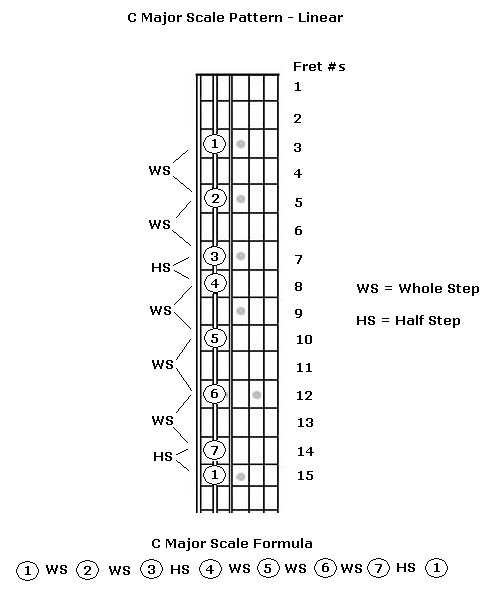C Major Scale for Beginners: Lesson 1

What is the C major scale?

A scale is a very specific pattern of notes that is used to create the basic building blocks of music: melody and harmony. In order to build a scale from the intervals of the octave we need a formula. The major scale formula is derived from the nature of the overtone series and is:

1st note, whole step, 2nd note, whole step, 3rd note, half step, 4th note, whole step, 5th note, whole step, 6th note, whole step, 7th note, half step, 8th note (which is one octave higher than the first note).

This formula produces the very familiar sound we associate with the solfege syllables: Do, Re, Mi, Fa, Sol, La, Ti, Do. Let's use this idea to play a C major scale. We start with the note C on the A string, 3rd fret. Therefore the note C is the 1st note of the scale (the "1st scale degree"). We move up a whole step (or two half steps) to the 5th fret and this will be the 2nd note of the C major scale (the "2nd scale degree"). This note happens to be a D. Follow the scale formula and we wind up with this pattern of notes:

C - whole step - D - whole step - E - half step - F - whole step - G - whole step - A - whole step - B - half step - C

On a guitar this can be played as such (see image below):

Open In New Window